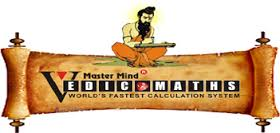# VEDIC MATHS TRICK TO FIND SQUARES OF NUMBERS EASILYTRICK TO FIND SQUARES OF NUMBERS ENDING WITH 5,9,1

Square of numbers ending with 5 Formula for calculating square ending with 5 is easy.

$\begin{array} { l l } & 85 \\ \times & 85 \\ \hline \\ & 7225 \end{array}$

Steps

• Multiply 5 by 5 and put composite digit 25 on the right hand side.
• Add 1 to the upper left hand side digit i.e. 8 i.e. 8+1=9
• Multiply 9 to the lower hand digit 8, i.e. 9*8=72

Using this method we can find out square of the number. Now let’s have a look at method of calculating square of adjacent number.

Forward Method
We know method to find square of a number ending with 5., say Square of 75=5625, then just have a look to find square of 76.
75’s square=5625(known)
76’square=75’square+ (75+76) =5625+151=5776.
So square of 76 is 5776.

Steps

• Steps are simple. The format shown above is self explanatory. But still I am explaining it.
• 75’square=5625 is known
• Add (75+76=151) to this to get 76’square
• 76’square=5776.

Reverse Method
As like forward method for calculating square of number which is 1 more than the given number whose square is known, we have reverse method to find square.
Now let me explain in detail the Reverse approach through which You will able to find out squares of a number which is one less than given number.
Consider the following example:
Suppose we know square of a number, say, 70; how to find square of 69?
(70)’square=4900(known)
(69)’square=4900-(69+70) =4900-139
=4761.6 years ago

This discussion board is a place to discuss our Daily Challenges and the math and science related to those challenges. Explanations are more than just a solution — they should explain the steps and thinking strategies that you used to obtain the solution. Comments should further the discussion of math and science.

When posting on Brilliant:

• Use the emojis to react to an explanation, whether you're congratulating a job well done , or just really confused .
• Ask specific questions about the challenge or the steps in somebody's explanation. Well-posed questions can add a lot to the discussion, but posting "I don't understand!" doesn't help anyone.
• Try to contribute something new to the discussion, whether it is an extension, generalization or other idea related to the challenge.

MarkdownAppears as
*italics* or _italics_ italics
**bold** or __bold__ bold
- bulleted- list
• bulleted
• list
1. numbered2. list
1. numbered
2. list
Note: you must add a full line of space before and after lists for them to show up correctly
paragraph 1paragraph 2

paragraph 1

paragraph 2

[example link](https://brilliant.org)example link
> This is a quote
This is a quote
    # I indented these lines
# 4 spaces, and now they show
# up as a code block.

print "hello world"
# I indented these lines
# 4 spaces, and now they show
# up as a code block.

print "hello world"
MathAppears as
Remember to wrap math in $$ ... $$ or $ ... $ to ensure proper formatting.
2 \times 3 $2 \times 3$
2^{34} $2^{34}$
a_{i-1} $a_{i-1}$
\frac{2}{3} $\frac{2}{3}$
\sqrt{2} $\sqrt{2}$
\sum_{i=1}^3 $\sum_{i=1}^3$
\sin \theta $\sin \theta$
\boxed{123} $\boxed{123}$